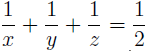# A note on a Diophantine equation

József Sándor
Notes on Number Theory and Discrete Mathematics, ISSN 1310-5132
Volume 19, 2013, Number 4, Pages 1—3

## Details

### Authors and affiliations

József SándorBabeș-Bolyai University, Department of Mathematics
Str. Kogălniceanu nr. 1, 400084 Cluj-Napoca, Romania

### Abstract

We offer an elementary approach to the solution of diophantine equationconsidered recently in Vol. 19, No. 3 of this journal. An extension is provided, too.

### Keywords

• Diophantine equations

• 11D68

### References

1. Rabago, J.F.T., R.P. Tagle. On the area and volume of a certain regular solid and the Diophantine equation 1/2 = 1/x + 1/y + 1/z . Notes on Number Theory and Discrete Mathematics, Vol. 19, 2013, No. 3, 28–32.
2. Zelator, K. An ancient Egyptian problem: The diophantine equation 4/n = 1/x + 1/y + 1/z (preprint).

## Cite this paper

APA

Sándor, J. (2013). A note on a Diophantine equation, Notes on Number Theory and Discrete Mathematics, 19(4), 1-3.

Chicago

Sándor, József. “A Note on a Diophantine equation.” Notes on Number Theory and Discrete Mathematics 19, no. 4 (2013): 1-3.

MLA

Sándor, József. “A Note on a Diophantine equation.” Notes on Number Theory and Discrete Mathematics 19.4 (2013): 1-3. Print.